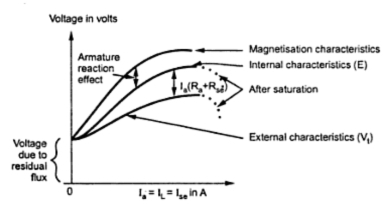### Load Characteristics of D.C. Series Generator

Consider a series generator shown in the Fig. 1

In case of series generator,
Ia = Ise =  IL
As load current increases,  Ise increases. The flux Φ is directly proportional to. So flux also increases. The induced e.m.f. E is proportional to  Ise flux hence induced e.m.f. also increases. Thus the characteristics of E against i.e. internal characteristics is of increasing nature. As increases Ia, armature reaction increases but its effect is negligible compared to increase in E. But for high load current, saturation occurs and flux remains constant. In such case, due to the armature reaction E starts decreasing as shown by dotted line in the Fig. 2.Fig. 1 Characteristics of d.c. series motor

Now as IL = I increases, thus the drop I (R +Rse) increases.
Vt = E - I (R +Rse)
Thus the external characteristics is also of rising nature as E increases but it will be below internal characteristics due to drop I (R +Rse). This is shown in the Fig. 2.
In self excited series generator, open circuit characteristics can not be obtained. In open circuit, I = IL = 0 hence induced e.m.f. is zero. Thus open circuit characteristics is possible only by separately exciting the field winding. It is also shown in the Fig. 2.
In practice when there is no load IL = 0, then there exists certain induced e.m.f. due to residual flux retained by the field winding.
Note : Hence for zero load current, there is a voltage present and the internal and external characteristics do not pass through origin as shown in the Fig. 2.